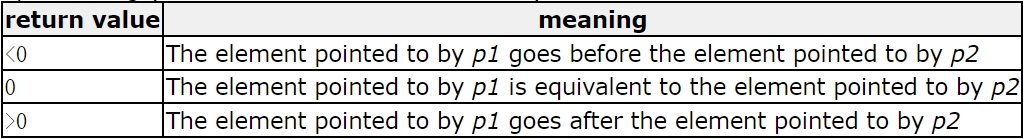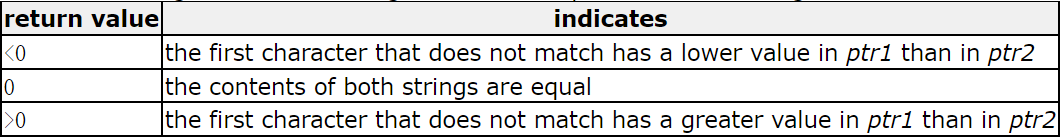##### 回调函数

``````void menu()
{
printf("#####################\n");
printf("###3.Mul     4.Div###\n");
printf("#####################\n");
}
{
return x + y;
}
int Sub(int x, int y)
{
return x - y;
}
int Mul(int x, int y)
{
return x * y;
}
int Div(int x, int y)
{
return x / y;
}
void Calc(int (*pf)(int ,int))
{
int a = 0;
int b = 0;
printf("输入两个操作数:>");
scanf("%d %d", &a, &b);
printf("%d\n", pf(a, b));
}

int main()
{
int input = 0;
do
{
printf("请选择:>");
scanf("%d", &input);
switch (input)
{
case 1:
break;
case 2:
Calc(Sub);
break;
case 3:
Calc(Mul);
break;
case 4:
Calc(Div);
break;
case 0:
printf("退出\n");
default:
printf("选择错误\n");
break;
}
} while (input);
return 0;
}``````

``````void Print(char* str)
{
printf("hello %s", str);
}
void test (void(*p)(char*))
{
p("world");  //p调用函数时可以解引用也可以不解引用
}
int main()
{
test(Print);
return 0;
}``````
``hello world``

###### 无类型指针void*

void*类型的指针可以接收任意类型的地址。 如下代码都不会提示警告：

``````int main()
{
int a = 10;
int* pa = &a;
char b = '1';
void* c = &pa;
void* d = &b;
return 0;
}    ``````

void*类型的指针不能进行解引用操作。 原因是void没有具体类型，无法得知解引用后可以操作的字节数。 ::: tip 报错：未知大小的指针。 ::: void类型的指针不能进行加减整数的运算。

##### qsort

::: tip qsort函数：

``````void qsort (void* base,
size_t num,
size_t size,
int (*compar)(const void*,const void*));``````

void * base：待排序的数组的首元素的指针，使用void*接收。 size_t num：base指向的数组中的元素个数。（size_t表示无符号整数类型） size_t size：数组中每个元素的大小（以字节为单位）。（size_t表示无符号整数类型） compar：比较两个元素的函数的指针。（使用者自己定义） qsort重复调用此函数以比较两个元素。该函数原型：

int compar (const void* p1, const void* p2); :::

qsort排序不同类型的数据时，因为数据的类型不同，需要的排序方法不同，所以需要使用cmp的指针传递要比较的两个元素的地址（e1、e2），传递元素地址时因为元素类型未知，使用void，传递到cmp_int函数（比较两个整型大小的函数）中使用void 接收任意类型的数据的地址。 在cmp_int函数中，e1和e2是两个void* 类型指针，比较大小时需要先强制类型转换成int*，然后再解引用得到值进行比较。 ::: tip qsort比较大小时的要求：e1<e2，返回<0数字；e1=e2，返回0；e1>e2，返回>0数字。 ::: 当使用qsort对结构体进行排序时，两个结构体对象是复杂对象，不能直接使用比较运算符进行比较，需要先确定排序时结构体中某一元素做参照物，如年龄等。如果使用年龄比较，相当于字符串比较，使用strcmp函数。strcmp比较字符串时，首字符排序在后的大。 ::: tip strcmp的函数返回值和qsort一样，如下：:::

##### qsort应用
``````#include <stdlib.h>
#include <string.h>

void bubble_sort(int arr[], int sz)
{
int i = 0;  //趟数
for (i = 0; i < sz - 1; i++)
{
//一趟冒泡排序
int j = 0;
for (j = 0; j < sz - 1 - i; j++)
{
if (arr[j] > arr[j + 1])
{
int tmp = arr[j];
arr[j] = arr[j + 1];
arr[j + 1] = tmp;

}
}
}
}

struct Stu
{
char name;
int age;
};

int cmp_int(const void* e1, const void* e2)
{
return *(int*)e1 - *(int*)e2;
//比较两个整型值时需要强制类型转换，void* 不可以解引用
}

void test1()
{
int arr = { 9,8,7,6,5,4,3,2,1,0 };
int sz = sizeof(arr) / sizeof(arr);
qsort(arr, sz, sizeof(arr), cmp_int);
int i = 0;
for (i = 0; i < sz; i++)
{
printf("%d ", arr[i]);
}
}

int cmp_float(const void* e1, const void* e2)
{
return ((int)(*(float*)e1 - *(float*)e2));
//(*(float*)e1 - *(float*)e2)相减结果再强制类型转换为int
}

void test2()
{
float f[] = { 9.0,8.0,7.0,6.0,5.0,4.0,3.0,2.0,1.0 };
int sz = sizeof(f) / sizeof(f);
qsort(f, sz, sizeof(f), cmp_float);
int j = 0;
for (j = 0; j < sz; j++)
{
printf("%f ", f[j]);
}
}

int cmp_stu_by_age(const void* e1, const void* e2)
{
return ((struct Stu*)e1)->age - ((struct Stu*)e2)->age;
}

int cmp_stu_by_name(const void* e1, const void* e2)
{
return strcmp(((struct Stu*)e1)->name, ((struct Stu*)e2)->name);
}

void test3()
{
struct Stu s = { {"zhangsan",20},{"lisi",22},{"wangwu",19} };
int sz = sizeof(s) / sizeof(s);
qsort(s, sz, sizeof(s), cmp_stu_by_age);
qsort(s, sz, sizeof(s), cmp_stu_by_name);
}

int main()
{
test1();
printf("\n");
test2();
test3();
return 0;
}``````

##### 可以接收任意类型数据的冒泡排序
``````int cmp_int(const void* e1, const void* e2)
{
return *(int*)e1 - *(int*)e2;
//比较两个整型值时需要强制类型转换，void* 不可以解引用
}
void Swap(char* buf1, char* buf2, int width)
{
int i = 0;
for (i = 0; i < width; i++)
{
int tmp = *buf1;
*buf1 = *buf2;
*buf2 = tmp;
buf1++;
buf2++;
}
}
//使用bubble_sort的程序员不知道未来要排序的数据的类型和待比较的两个元素的类型，写void*
void bubble_sort(void* base, int sz, int width,int (*cmp)(void* e1,void* e2))
{
int i = 0;  //趟数
for (i = 0; i < sz; i++)
{
int j = 0;  //每一趟比较的元素对数（每一对比较两个元素）
for (j = 0; j < sz - 1 - i; j++)
{
//使用compar函数比较两个元素,需要传入两个元素的地址
if (cmp((char*)base + j * width, (char*)base + (j + 1) * width) > 0)  //升序排序比较结果>0交换
{
Swap((char*)base + j * width, (char*)base + (j + 1) * width,width);
//元素交换时是一个元素一个元素的交换，需要传入width表示需要交换几对元素
}
}
}
}

void test4()
{
int arr = { 9,8,7,6,5,4,3,2,1,0 };
int sz = sizeof(arr) / sizeof(arr);
//使用bubble_sort的程序员一定知道要排序的数据类型和比较方法
bubble_sort(arr,sz,sizeof(arr),cmp_int);
int i = 0;
for (i = 0; i < sz; i++)
{
printf("%d ", arr[i]);
}
}

struct Stu
{
char name;
int age;
};

int cmp_stu_by_age(const void* e1, const void* e2)
{
return ((struct Stu*)e1)->age - ((struct Stu*)e2)->age;
//强制类型转换成结构体指针形式
}

int cmp_stu_by_name(const void* e1, const void* e2)
{
return strcmp(((struct Stu*)e1)->name, ((struct Stu*)e2)->name);
}

void test5()
{
struct Stu s = { {"zhangsan",20},{"lisi",22},{"wangwu",19} };
int sz = sizeof(s) / sizeof(s);
//bubble_sort(s, sz, sizeof(s), cmp_stu_by_age);
bubble_sort(s, sz, sizeof(s), cmp_stu_by_name);
}

int main()
{
//test4();
printf("\n");
test5();
return 0;
}``````Suzhou
Lv1

17

9

0